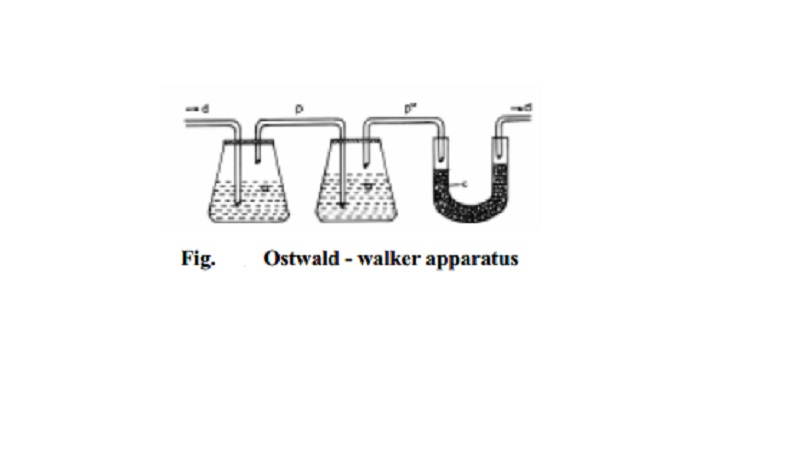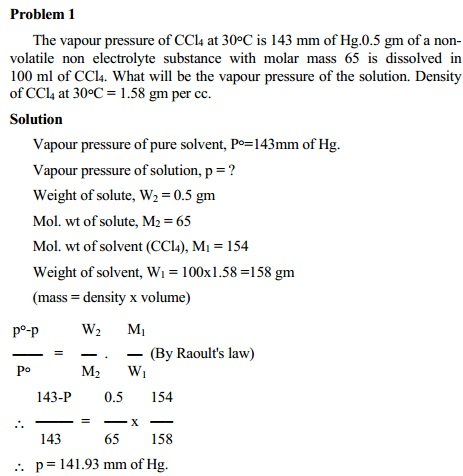Home | | Chemistry | Raoult's Law and Dynamic method (or) Ostwald - Walker method

# Raoult's Law and Dynamic method (or) Ostwald - Walker methodThe relationship between the vapour pressure of the solution and its concentration is given by a French chemist named Francois Marie Raoult (1886). According to Raoult's law, at constant temperature the vapour pressure of the solution (p) is directly proportional to the molefraction of the solvent (X1) present in the solution.

Raoult's Law

The relationship between the vapour pressure of the solution and its concentration is given by a French chemist named Francois Marie Raoult (1886). According to Raoult's law, at constant temperature the vapour pressure of the solution (p) is directly proportional to the molefraction of the solvent (X1) present in the solution. That is, p . ;1 (or) p = kX1 where k is the proportionality constant. The value of k is known as follows: For a pure solvent, X1 = 1.0 and p becomes p o corresponding to the vapour pressure of the pure solvent. Thus, p o = k (1.0). Substituting the value of k,

p = p o X1      -------  1

Equation 1 is generally known as Raoult's law.

When n1 and n2 are the number of moles of solvent and solute present in the solution, the molefraction of the solvent X1 = n1/(n1 + n2) and the mole fraction of solute X2 = n2/(n1+n2). Also, X1 + X2 = 1.0

If W1 and W2 are the weights of solvent and solute present, then n1 = W1/M1 and n2 = W2/M2. M1 and M2 are the molar masses of solvent and solute respectively.

It is generally observed that p is lower than P o . The lowering of vapour pressure of the solvent in the solution equals to (p o - �S

The relative lowering of the vapour pressure, is defined as the ratio of the lowering of vapour pressure to the vapour pressure of the pure solvent. Thus relative lowering of vapour pressure is given by

p o -p /P o   =  ∆P / P o

p o -p / p o   = p o -p o X1 / p o     since p = p o X1

P o (1-X1) / P o = 1-X1 = X2.... since X1+X2 = 1

{ }

p o -p /  p o =  X2   ------- 2

Equation 2 represents the mathematical from of Raoults law. Thus, the statement of Raoult's law for dilute solutions containing non-volatile non-electrolyte solute is: Relative lowering of vapour pressure is equal to the mole fraction of the solute. Since mole fraction of the solute (X2) is given by n2/(n1+n2), the quantity (p-p)/p depends upon the number of moles or molecules of the solute in solution and not on its chemical nature. Thus, relative lowering of vapour pressure is a colligative property.

Determination of molecular weights from relative lowering of vapour pressure

In dilute solutions, the number of moles of solvent (n1) is large compared to the number of moles of solute (n2) and thus (n1 + n2) can be approximated to n1 and x2 becomes equal to n2/n1.

Thus ∆p /p0 =   n2 / n1 =  W2.M1 / M2.W1     ---------3

Substituting   for n1 and   n2 as   W1/M1    and W2/M2 we get

∆p /p0 = M1.W2/W1.M2. Knowing M1,W1   and  W2 and from the measurement of lowering of vapour pressure, M2 the molar mass of the solute can be determined using equation.3.Dynamic method (or) Ostwald - Walker method

This method is based on the principle that when dry air is successively passed through a series of containers possessing solution and pure solvent respectively, the air becomes saturated with the solvent vapours and an equal amount of weight loss in solution and solvent containers takes place.

In Fig. the first chamber (a) contains a weighed amount of the solution under examination and the next chamber (b) contains a weighed amount of the pure solvent. A weighed amount of anhydrous and dry calcium chloride is taken in the U-tube (c) connected at the end. The chambers and the U-tube are connected by a series of delivery tubes(d) through which air is passed. The dry air is first allowed to pass through the solution chamber until the air is saturated with the solvent vapour to maintain the vapour pressure of the solution `p'. Consequently, a loss in weight of the solution results in the solution chamber since some amount solvent molecules have evaporated. When this air is allowed to pass through the pure solvent chamber some more solvent vapour gets in stream with air, until the vapour pressure of pure solvent p o , is maintained. This happens so because p o is greater than p. Consequently, the weight loss registered in the solvent chamber is proportional to the (p o -p) quantity.

The weight loss in solution chamber . S

The weight loss in solvent chamber  . S o -p

Sum of the loss in weights of solution

and solvent chamber             . (p+p o -p) . S o

When the air saturated with solvent vapours is passed through CaCl2 U-tube, the solvent vapours are absorbed and the dry air gets out. The gain in weight of the CaCl2 U-tube should be equal to the total loss in weight of solution and solvent chambers, which is inturn proportional to p o .

Loss in weight of the solvent chamber / Gain in weight of CaCl2 tube = p o -p  /  p o

= relative lowering of the vapour pressure

Thus, using the experimental (p o -p)/p o values and applying Raoult's law, the molecular weight of the solute can be determined.Study Material, Lecturing Notes, Assignment, Reference, Wiki description explanation, brief detail
11th 12th std standard Class Organic Inorganic Physical Chemistry Higher secondary school College Notes : Raoult's Law and Dynamic method (or) Ostwald - Walker method |